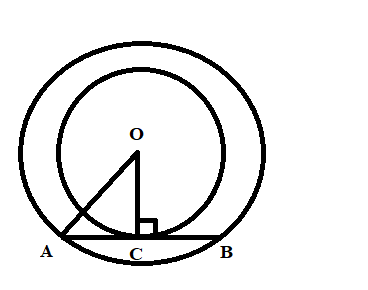QUESTION

# Two concentric circles of radii a and b, where a > b, are given. The length of the chord of the larger circle which touches the smaller circle is:a. $\sqrt{{{a}^{2}}-{{b}^{2}}}$b. $\sqrt{{{a}^{2}}+{{b}^{2}}}$c. $2\sqrt{{{a}^{2}}-{{b}^{2}}}$d. $2\sqrt{{{a}^{2}}+{{b}^{2}}}$

Hint: By using the theorem “The tangent and radius at point of contact are perpendicular”, the angle made by the radius of small circle with one of the end point of the chord which touches the larger circle is ${{90}^{\circ }}$. Applying the Pythagoras theorem in the obtained triangle, we get the length of half chord. After applying the theorem “The perpendicular drawn from the center bisect the chord”, we get the length of the chord. Using this methodology, we can easily solve the problem.

Complete Step-by-Step solution:We draw two circles having center O. The radius of the smaller circle is OC and the radius of the larger circle is OA. AB is the chord in a larger circle which touches a smaller circle at a point C. Now, we have to find the length of AB.
$\angle OEC={{90}^{\circ }}$ (Using the theorem “The tangent and radius at the point of contact are perpendicular”)
As stated by Pythagoras, In the right-angle triangle, the sum of squares of hypotenuse is equal to the sum of squares of both other sides. Now, applying the Pythagoras theorem in the triangle AOC, we get
\begin{align} & A{{O}^{2}}=O{{C}^{2}}+A{{C}^{2}} \\ & {{a}^{2}}={{b}^{2}}+A{{C}^{2}} \\ & AC=\sqrt{{{a}^{2}}-{{b}^{2}}} \\ \end{align}
Now, by using the theorem “the perpendicular drawn from the center of the circle bisects the chord”, we can express AB as, AB = 2AC.
$AB=2\sqrt{{{a}^{2}}-{{b}^{2}}}$
Hence, the length of the chord is $2\sqrt{{{a}^{2}}-{{b}^{2}}}$.
Therefore, option (c) is correct.

Note: The key concept in solving this problem is the knowledge of theorems related to circles. By using various properties, we obtained our answer without any error. This knowledge is helpful in solving complex problems.